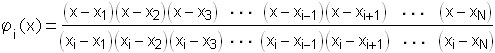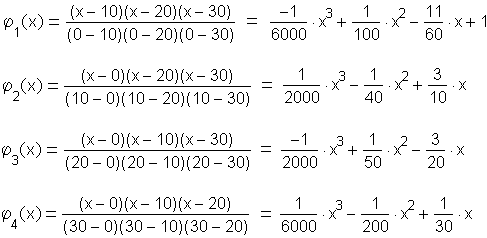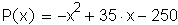Lagrange Interpolation Polynomials

If we wish to describe all of the ups and downs in a data set, and hit every point, we use what is called an interpolation polynomial. This method is due to Lagrange.

Suppose the data set consists of  N  data points:

(x1, y1), (x2, y2), (x3, y3), ..., (xN, yN)

The interpolation polynomial will have degree  N – 1 . It is given by:

P(x) = j1(x) y1 + j2(x) y2 + j3(x) y3 + ... + jN(x) yN

where the functions  ji(x)  (i = 1, 2, 3, ..., n)  are given by:Don't fret! This is easier than it looks. The key thing to notice is that the numerator of  ji(x)  contains the entire sequence of factors  (x – x1), (x – x2), (x – x3), ... (x – xN), with the exception of the single factor  (x – xi). Likewise, the denominator contains the entire sequence of factors  (xi – x1), (xi – x2), (xi – x3), ... (xi – xN), with the exception of the single factor  (xi – xi).

Now notice:  ji(xi) = 1  (numerator = denominator), but  ji(xj) = 0  (numerator = 0, since it contains the factor  (xj – xj) ) for any  j  not equal to  i . This means that  P(xi) = yi , which is exactly what we want!

If this seems like smoke and mirrors, consider a simple example. Here's the data for  g  again:

 x g(x) 0 –250 10 0 20 50 30 –100

In this case there are  N = 4  data points, so we will create a polynomial of degree  N – 1 = 3 . We have  x1 = 0 ,  x2 = 10 ,  x3 = 20 ,  x4 = 30 , and  y1 = –250 ,  y2 = 0 ,  y3 = 50 ,  y4 = 100 . So:Multiplying each of these by the appropriate  yi   (i = 1, 2, 3, 4)  and adding together the terms of like power then gives:The cubic terms cancel, and we arrive at a simple quadratic description of the data.

A quick plot of the data together with the polynomial shows that it indeed passes through each of the data points:For an interactive demonstration of Lagrange interpolation polynomials, showing how variations in the data points affect the resulting curve, go here.